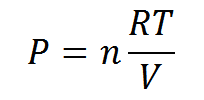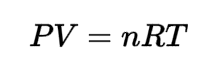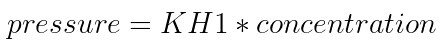Request a Tool

# Partial Pressure

The pressure that would be applied by one of the gases in a mixture if it absorbed the same volume on its own.

Partial Pressure
0

Partial Pressure
0

Partial Pressure
0

#### Formula• P = Pressure of the gas
• V = Volume
• n = moles of gas
• T = Temperature
• R = 8.314 J K-1 mol-1, ideal gas constant.

#### Defination / Uses

Dalton's law of partial pressure measures total pressure using temperature, moles of gas and volume.

#### Formula• P = Pressure of the gas
• V = Volume
• n = moles of gas
• T = Temperature
• R = 8.314 J K-1 mol-1, ideal gas constant.

#### Defination / Uses

The ideal gas law is the equation of state of a hypothetical ideal gas. It is a good approximation of the behaviour of many gases under many conditions, although it has several limitations.

### Uses

ideal gas law can be used to calculate Temperature, Pressure,Volume and Moles of gases consumed or produced.

#### Formula• KH1 = Henry's law constant in [litre*atm/mol].

#### Defination / Uses

Henry's law is only accurate at low gas pressures ( pressures< 1000 hPa), constant temperatures ( generally 293.15 K) and when the motes are at equilibrium.

We measure gas pressure in daily life when we use a barometer to check the outside atmospheric pressure or a tires gauge to check the pressure in a bike tube. We're measuring a macroscopic physical property of a large number of gas molecules which aren't visible to the human eye when we perform this. The pressure we're measuring is the product of specific gas molecules colliding with other objects, such as the container's walls, at the molecular level.

This ideal gas law calculator can help to determine the parameters of an ideal gas as a result of changes in pressure, temperature, or volume. Continue reading to learn about the properties of an ideal gas, how to utilise the ideal gas law equation, and how to calculate the ideal gas constant. An ideal gas is a hypothetical gas made up by scientists and students because the Ideal Gas Law would be lot simpler if things like intermolecular forces didn't exist to complicate it. Ideal gases are simply point masses travelling in a straight line at a constant, random speed. The assumptions given in the Kinetic-Molecular Theory of Gases characterise its behaviour. Use the upper given formula for manual calculations. No sign-up, registration OR captcha is required to use this tool.